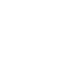## 大型工业风扇通风降温的原理1、直径 730mm 的风扇：周长=0.73 × 3.14=2 .29m 截面积=0.36 × 0.36 × 3.14=0.407 平方米

2、直径 7200mm 的风扇 ：周长=7.2 × 3.14=22.9m 截面积=3.6 × 3.6 × 3.14=40.7 平方米

1、直径 730mm 的风扇：周长=0.73 × 3.14=2 .29m 截面积=0.36 × 0.36 × 3.14=0.407 平方米

2、直径 7200mm 的风扇 ：周长=7.2 × 3.14=22.9m 截面积=3.6 × 3.6 × 3.14=40.7 平方米

### 相关新闻